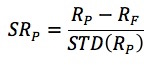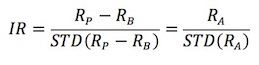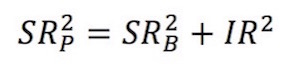### Seeing is believing!

Before you order, simply sign up for a free user account and in seconds you'll be experiencing the best in CFA exam preparation.

##### Subject 2. Comparing Risk and Return
The Sharpe Ratio

The Sharpe ratio measures the reward to total volatility trade-off.• RF: the risk-free rate
• STD(RP): the standard deviation of the portfolio returns

The Sharpe ratio is also called the reward-to-variability ratio. Portfolios with large Sharpe ratios are preferred to those with smaller ratios because we assume that investors prefer returns and dislike risk.

The Sharpe ratio can be used as an ex ante measure of expected return and risk or an ex post performance of a portfolio over some time period.

Example

The mean monthly return on T-bills (the risk-free rate) is 0.25%. The mean monthly return on the S&P 500 is 1.30% with a standard deviation of 7.30%.

Sharpe ratio = (1.30 - 0.25)/7.3 = 0.144

The convention is to annualize both the return and standard deviation.

Sharpe ratio = (12 x 1.30 - 12 x 0.25)/(7.3 x 121/2) = 0.498

The Sharpe ratio is unaffected by the addition of cash or leverage in a portfolio. An investor should form portfolios with two funds: one is the risk-free asset and the other is the risky portfolio with the highest Sharpe ratio. The investor can then adjust risk and return based on his/her preference.

The Information Ratio

While the Sharpe ratio measures reward per unit of risk in absolute returns, the information ratio measures reward per unit of risk in benchmark relative returns.where active return = RP - RB = RA and active risk = STD(RA).

Comparisons:

• Both ratios can be applied ex ante to expected returns or ex post to realized returns.
• Higher information ratio portfolios can be used to create higher Sharpe ratio portfolios.
• The Sharpe ratio compares the return of an asset against the return of Treasury bills; the information ratio compares excess return to the most relevant equity (or debt) benchmark index. The information ratio helps investors focus on the relative value added by active management.
• Unlike the Sharpe ratio, the information ratio is affected by the addition of cash or the use of leverage.

The information ratio will remain unchanged if all the active security weights are multiplied by a constant. This is because both the active return and active risk increase proportionally.

Constructing Optimal Portfolios

Note the LOS requires that you "calculate and interpret the information ratio and contrast it to the Sharpe ratio." This section is not required.

With a risk-free asset and a single risky portfolio with the maximum Sharpe ratio, we can adjust absolute risk and return of our portfolio.

With an actively managed portfolio and the benchmark portfolio, we can adjust active risk and return of our portfolio.This implies that the higher the information ratio, the higher the Sharpe ratio. The information ratio is a key criterion on which to evaluate actively managed portfolios.

The optimal amount of active risk that maximizes the Sharpe ratio but keeps the same information ratio can be determined by:Once the optimal level of active risk is determined, the actively managed portfolio can be combined with a position in the benchmark portfolio to maximize the Sharpe ratio.

User Comment
thebkr777 How sure are we that we do not need to calculate this?
jimmyvo We do need to calculate. LOS says, "Calculate and interpret..."